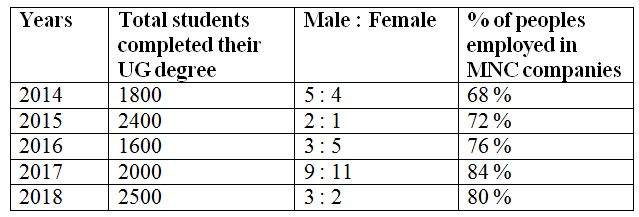# IBPS RRB PO Prelims Quantitative Aptitude Questions 2019 (Day-05)

Dear Aspirants, Our IBPS Guide team is providing new series of Quantitative Aptitude Questions for IBPS RRB PO Prelims 2019 so the aspirants can practice it on a daily basis. These questions are framed by our skilled experts after understanding your needs thoroughly. Aspirants can practice these new series questions daily to familiarize with the exact exam pattern and make your preparation effective.

Check here for IBPS RRB Prelims Mock Test 2019

[WpProQuiz 6594]

Directions (Q. 1 – 5): What value should come in place of (?) in the following questions?

1) 18 % of 3600 + 24 % of 1200 – ? = 30 % of 1200

a) 576

b) 624

c) 692

d) 458

e) None of these

2) 864 ÷ (12 % of 450) = 14 × 3 5/7 of (? ÷ 13)

a) 8

b) 12

c) 4

d) 16

e) None of these

3) (35200 ÷ 11) + (2/9) of 31590 + 152 = 25 % of ?

a) 41780

b) 37820

c) 43540

d) 39660

e) None of these

4) 729 × 11 ÷ 27 – 15 % of 2000 = ? – 133

a) 2872

b) 2194

c) 2658

d) 2466

e) None of these

5) 234.75 + 1125.25 + 12 % of 1200 = ? + 11 × 15

a) 1575

b) 1847

c) 1339

d) 2068

e) None of these

Directions (Q. 6 – 10): Study the following information carefully and answer the given questions?

The following table shows the total number of students completed their UG degree in different years in a certain college and the ratio of male and female. The percentage of peoples employed in MNC companies and the remaining peoples are doing own business.6) Find the ratio between the total number of students employed in MNC companies in the year 2014 and the total number of peoples who are doing business in 2018?

a) 155 : 79

b) 217 : 113

c) 346 : 251

d) 52 : 15

e) None of these

7) Find the average number of students who completed their UG degree in all the given years together?

a) 1820

b) 1640

c) 2280

d) 2060

e) None of these

8) Find the difference between the total number of males who completed their degree in the year 2015 to that of total number of females who completed their degree in the year 2017?

a) 500

b) 750

c) 600

d) 900

e) None of these

9) The total number of peoples employed in MNC companies in the year 2015 is approximately what percentage of total number of peoples employed in MNC companies in the year 2017?

a) 126 %

b) 88 %

c) 103 %

d) 147 %

e) 165 %

10) The total number of peoples who completed their UG degree in 2016 and doing business is what percentage more/less than the total number of female students who completed their UG degree in the year 2014?

a) 36 % more

b) 52 % less

c) 36 % less

d) 52 % more

e) 64 % more

Direction (1-5) :

(18/100)*3600 + (24/100)*1200 – x = (30/100)*1200

648 + 288 – 360 = x

x = 576

864 ÷ (12 % of 450) = 14 × 3 5/7 of (x ÷ 13)

864 ÷ [(12 / 100) * 450] = 14 * (26/7)*(x/13)

864 ÷ 54 = 4x

16 = 4x

x = 16/4 = 4

(35200/11) + (2/9)*31590 + 152 = (25/100)*x

3200 + 7020 + 225 = (25/100)*x

10445*(100/25) = x

x = 41780

(729*11)/27 – (15/100)*2000 + 2197 = x

297 – 300 + 2197 = x

x = 2194

234.75 + 1125.25 + (12/100)*1200 – 11 × 15 = x

234.75 + 1125.25 + 144 – 165 = x

x = 1339

Direction (6-10) :

The total number of students employed in MNC companies in the year 2014

= > 1800*(68/100)

The total number of students who completed their UG degree in 2018 and doing business

= > 2500*(20/100)

Required ratio = [1800*(68/100)] : [2500*(20/100)]

= > 306 : 125

The average number of students who completed their UG degree in all the given years together

= > (1800 + 2400 + 1600 + 2000 + 2500) / 5

= > (10300/5) = 2060

The total number of males who completed their degree in the year 2015

= > 2400*(2/3) = 1600

The total number of females who completed their degree in the year 2017

= > 2000*(11/20) = 1100

Required difference = 1600 – 1100 = 500

The total number of peoples employed in MNC companies in the year 2015

= > 2400*(72/100) = 1728

The total number of peoples employed in MNC companies in the year 2017

= > 2000*(84/100) = 1680

Required % = (1728/1680)*100 = 102.85 % = 103 %

The total number of peoples who completed their UG degree in 2016 and doing business

= > 1600*(24/100) = 384

The total number of female students who completed their UG degree in the year 2014

= > 1800*(4/9) = 800

Required % = [(800 – 384) / 800] * 100 = 52 % less